Courses

# Test: Calculations, Exponents And Basic Algebra

## 10 Questions MCQ Test Quantitative Aptitude for GMAT | Test: Calculations, Exponents And Basic Algebra

Description
This mock test of Test: Calculations, Exponents And Basic Algebra for GMAT helps you for every GMAT entrance exam. This contains 10 Multiple Choice Questions for GMAT Test: Calculations, Exponents And Basic Algebra (mcq) to study with solutions a complete question bank. The solved questions answers in this Test: Calculations, Exponents And Basic Algebra quiz give you a good mix of easy questions and tough questions. GMAT students definitely take this Test: Calculations, Exponents And Basic Algebra exercise for a better result in the exam. You can find other Test: Calculations, Exponents And Basic Algebra extra questions, long questions & short questions for GMAT on EduRev as well by searching above.
QUESTION: 1

###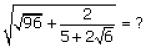lies between:

Solution:

The first culprit in this expression is the radical in the denominator. Radicals in the denominator are dealt with by multiplying the fraction with an expression that is equal to 1 but contains a “canceling radical” in both the numerator and denominator.
For example,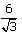is simplified by multiplying by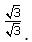In the case of a complex radical, such as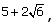we multiply by the conjugate,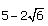as follows: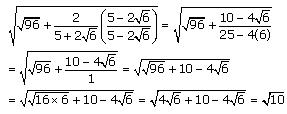Simplifying radicals in the denominator with conjugate radical expressions is very useful on challenging GMAT radical questions.

QUESTION: 2

###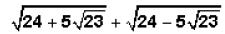lies between:

Solution:

In order to rid the expression of square roots, let's first square the entire expression. We are allowed to do this as long as we remember to "unsquare" whatever solution we get at that end.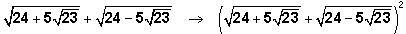Notice that the new expression is of the form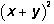where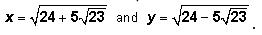Recall that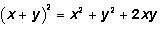This is one of the GMAT's favorite expressions.
Returning to our expression: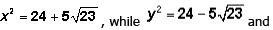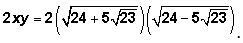Notice that x2 + y2  neatly simplifies to 48. This leaves only the 2xy expression left to simplify.
In order to simplify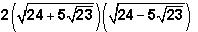recall that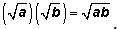Thus,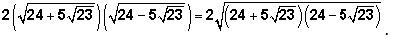Notice that the expression under the square root sign is of the form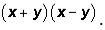And recall that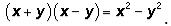This is another one of the GMAT's favorite expressions. Returning to our expression: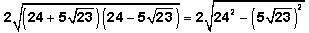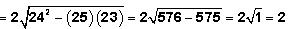Finally then: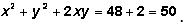But now we must remember to "unsquare" (or take the square root of) our answer: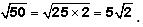QUESTION: 3

### A, B, C, D, E, F, G, and H are all integers, listed in order of increasing size. When these numbers are arranged on a number line, the distance between any two consecutive numbers is constant. If G and H are equal to 512 and 513, respectively, what is the value of A?

Solution: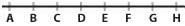The distance from G to H is 513 - 512.
The distance between and two consecutive points is constant, so the distance from A to G will be 6 times the distance from G to H or 6(513 – 512).
The value of A , therefore, will be equal to the value of G minus the distance from A to G :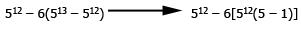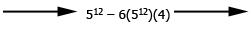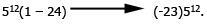QUESTION: 4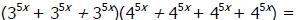Solution: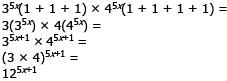Remember that when you multiply different bases raised to the SAME exponent, the product is simply the product of the bases raised to their common exponent.

QUESTION: 5

The three-digit positive integer x has the hundreds, tens, and units digits of a, b, and c, respectively. The three-digit positive integer y has the hundreds, tens, and units digits of k, l , and m, respectively. If (2a)(3b)(5c) = 12(2k)(3l)(5m), what is the value of x – y?

Solution:

First, let us simplify the exponential equation: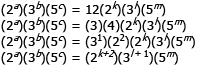When the bases on both sides of an equation are equal and the bases are prime numbers, the exponents of the respective bases must also be equal: a = k + 2; b = l + 1; and c = m . Now recall that a , b , and c represent the hundreds, tens, and units digits of the three-digit integer x ; similarly, k , l , and m represent the hundreds, tens, and units digits of the three-digit integer y .
Therefore, the hundreds digit of x is 2 greater than the hundreds digit of y ; the tens digit of x is 1 greater than the tens digit of y ; finally, the units digit of x is equal to the units digit of y . Using this information, we can set up our subtraction problem and find the value of (x – y ):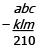QUESTION: 6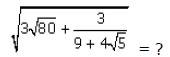Solution:

A radical expression in a denominator is considered non-standard. To eliminate a radical in the denominator, we can multiply both the numerator and the denominator by the conjugate of that denominator.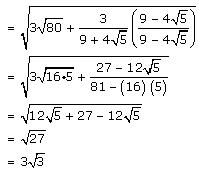QUESTION: 7

If 274x + 2 × 162-2x × 36x × 96 – 2x = 1, then what is the value of x?

Solution:

An effective strategy for problems involving exponents is to break the bases of all the exponents into prime factors. This technique will allow us to combine like terms:
274x + 2 × 162-2x × 36x × 96 – 2x = 1 (33)4x + 2 × (2 × 34)-2x × (22 × 32)x × (32)6 – 2x = 1
312x + 6 × 2-2x × 3-8x × 22x × 32x × 312 4x = 1
2-2x + 2x × 312x + 6 – 8x + 2x + 12 – 4x = 1
20 × 3 2x + 18 = 1
3 2x + 18 = 1
3 2x + 18 = 30
2x + 18 = 0
2x = -18
x = -9

QUESTION: 8

If (22x+1)(32y-1) = 8x27y, then x + y =

Solution:

Let's rewrite the right side of the equation in base 2 and base 3: (22x+1)(32y-1) = (23)x(33)y. This can be rewritten as: (22x+1)(32y-1) = 23x33y Since both bases on either side of the equation are prime, we can set the exponents of each respective base equal to one another: 2x + 1 = 3x , so x = 1 2y – 1 = 3y, so y = -1 Therefore, x + y = 1 + (-1) = 0.

QUESTION: 9

x2 + bx + 72 = 0 has two distinct integer roots; how many values are possible for 'b'?

Solution:

In quadratic equations of the form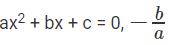represents the sum of the roots of the quadratic equation and c/a

represents the product of the roots of the quadratic equation.

In the equation given a = 1, b = b and c = 72

So, the product of roots of the quadratic equation = 72/1 = 72

And the sum of roots of this quadratic equation= -b/1 = -b

We have been asked to find the number of values that 'b' can take.
If we list all possible combinations for the roots of the quadratic equation, we can find out the number of values the sum of the roots of the quadratic equation can take.
Consequently, we will be able to find the number of values that 'b' can take.

The question states that the roots are integers.
If the roots are r1 and r2, then r1 * r2 = 72, where both r1 and r2 are integers.
Possible combinations of integers whose product equal 72 are : (1, 72), (2, 36), (3, 24), (4, 18), (6, 12) and (8, 9) where both r1 and r2 are positive. 6 combinations.

For each of these combinations, both r1 and r2 could be negative and their product will still be 72.
i.e., r1 and r2 can take the following values too : (-1, -72), (-2, -36), (-3, -24), (-4, -18), (-6, -12) and (-8, -9). 6 combinations.

Therefore, 12 combinations are possible where the product of r1 and r2 is 72.
Hence, 'b' will take 12 possible values.

QUESTION: 10

If x > 0, how many integer values of (x, y) will satisfy the equation 5x + 4|y| = 55?

Solution:

5x + 4|y| = 55
The equation can be rewritten as 4|y| = 55 - 5x.
Because |y| is non-negative, 4|y| will be non-negative. Therefore, (55 - 5x) cannot take negative values.

Because x and y are integers, 4|y| will be a multiple of 4.
Therefore, (55 - 5x) will also be a multiple of 4.
55 is a multiple of 5. 5x is a multiple of 5 for integer x. So, 55 - 5x will always be a multiple of 5 for any integer value of x.
So, 55 - 5x will be a multiple of 4 and 5.
i.e., 55 - 5x will be a multiple of 20.

Integer values of x > 0 that will satisfy the condition that (55 - 5x) is a multiple of 20:
1. x = 3, 55 - 5x = 55 - 15 = 40.
2. x = 7, 55 - 5x = 55 - 35 = 20
3. x = 11, 55 - 5x = 55 - 55 = 0.
When x = 15, (55 - 5x) = (55 - 75) = -20. Because (55 - 5x) has to non-negative x = 15 or values greater than 15 are not possible.
So, x can take only 3 values viz., 3, 7, and 11.

We have 3 possible values for 55 - 5x. So, we will have these 3 values possible for 4|y|.
Possibility 1: 4|y| = 40 or |y| = 10. So, y = 10 or -10.
Possibility 2: 4|y| = 20 or |y| = 5. So, y = 5 or -5.
Possibility 3: 4|y| = 0 or |y| = 0. So, y = 0.

Number of values possible for y = 5.

The correct choice is (C) and the correct answer is 5.